# Book Review: Passion for Mathematics by Clifford PickoverRecently, I came across the works of Clifford Pickover. I finished reading Surfing Through Hyperspace and I found it extremely interesting, and so I decided to try another book from his vault – Passion for Mathematics.

This book is equally fascinating. I liked the presentation of the content. Firstly, the various mathematical ideas are introduced with diagrams and puzzles, which helps in understanding the notion better.

Secondly, quotes are sprinkled here and there to give the subject some poetic effect and depth to abstract concepts.

Thirdly, the brain teasers and mazes were quite entertaining. After reading the book, I realised maths as a subject can be so much fun. Wow!

If you want to see the universe through the lens of numbers, just pick up this book, you won’t regret. Also, mathematics is not terrifying, the numbers are very friendly, trust me!

## Universe operates on mathematical laws

First section of the book answers some existential questions through the language of mathematics. The author reflects on the idea that universe operates on mathematical laws.

Pickover illustrates how for Pythagoras and his followers, numbers were like gods. Since they never altered, rather they were pure and free from material change. Therefore, they worshiped numbers 1 through 10. It was kind of polytheism for the Pythagoreans.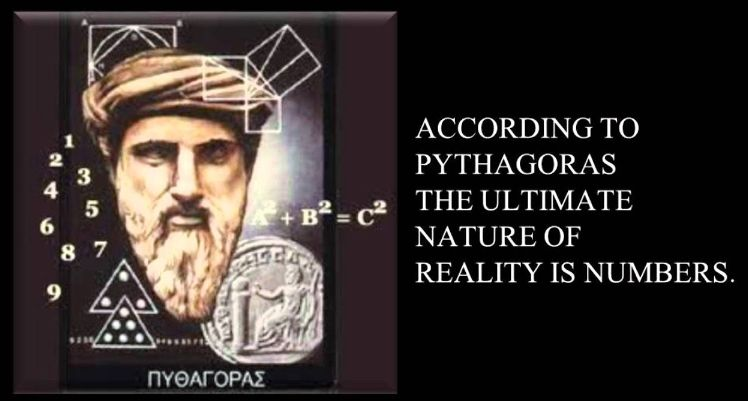## Mathematics is the fabric of reality

He believes that mathematics is the fabric of reality. The deeper we go in our understanding of the physical universe, the more we find that mathematical principles underlie everything. Be it quantum reality or string theory, every grand theory of the universe converges to the point of precise and quantitative terms of pure mathematics.

Even Einstein’s theories were not just philosophical musings but were also based on rigorous mathematical analysis and empirical testing.

## A tool for understanding reality

Mathematics is a complex and multifaceted subject that can be viewed from different perspectives. Whether seen as a tool for understanding the physical world or as a product of human creativity, it remains an essential part of human culture and knowledge.

Mathematicians and scientists are of the view that mathematical formulae have an inherent beauty and elegance that goes beyond their practical usefulness. All equations and formulas are embedded with intricate patterns and symmetries that reveal deep insights into the nature of the universe.

## Mathematical Platonism

So, what does the math reveal about reality? Pickover favours mathematical Platonism. According to which, all mathematical structures and equations are not just human inventions or thought neither perception but rather they exist as real entities that underlie and govern the physical world.

In this view, the energy flows through each particle of matter and energy. And this can be best described and understood through mathematical equations and structures.

Hence, entire reality that we experience is a manifestation of these underlying mathematical principles. The past, present, and future of the universe are seen as unfolding according to these mathematical laws.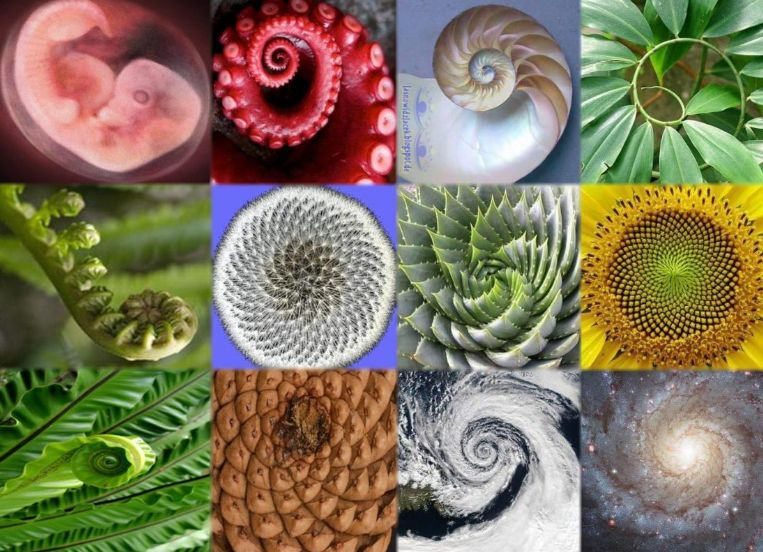## Nature is mathematics

The reality has a certain order and symmetry to the universe. And that can be explained through mathematical principles.

For instance, spirals found in sunflower heads and other flowers follow a pattern, scientists call it the Fibonacci numbers.

Fibonacci numbers follows a sequence as per which, each number is the sum of the two preceding numbers (0, 1, 1, 2, 3, 5, 8, 13, 21, etc.).

Interestingly, from the branching patterns of trees to the spirals of seashells and galaxies, every entity follows this order.

## Is God a mathematician?

So, the next obvious question that comes to our mind is, who created these laws? Or is God, the divine creator, a mathematician?

Pickover suggests that mathematics is a tool that “God” uses to bring order and structure to the natural world.

Accordingly, the laws of physics and the patterns including symmetries that we find in nature are the expressions of the mathematical principles that God has used to create and sustain the universe.

## Number sequences

Pickover also enumerates lots of fascinating and strange number sequences. He believes that mathematics is an ever-expanding field, hence it is soddened with countless number of concepts, theories, and discoveries waiting to be explored.

Accordingly, mathematical ideas are constantly moving and changing. Like bubbles in an ocean, every now and then new insights and breakthroughs are appearing and disappearing, on the surface of the water.

In a fascinating manner, the author suggests that one can only navigate in the ocean of mathematical knowledge by pushing the limitations that we know and dare to explore new areas of research and discovery.

## Algebra is the metaphysics of arithmetic

Pickover quotes John Ray statement, “Algebra is the metaphysics of arithmetic”.

Since, algebra is all about variables (unknown quantities that can take on different values), equations, and abstract concepts like functions, groups, rings, and fields; Arithmetic deals with concrete numbers and their manipulation.

Thus, suggesting that algebra is a more abstract and theoretical version of arithmetic.

An analogical interpretation could be that numbers acting as soldiers while algebra is supposed to set the rules of engagement. The techniques set forth by algebra could be used for manipulating and solving equations. Thus, allowing us to exercise the power of numbers with greater precision and effectiveness.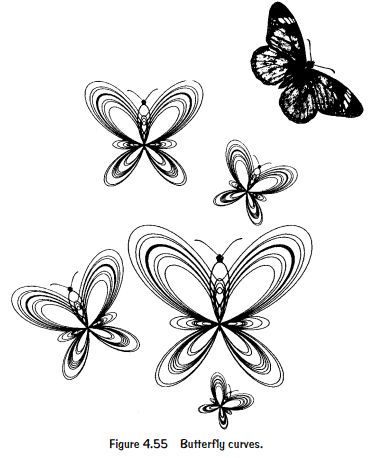## Geometry is silly putty of mathematics

Pickover, in the subsequent part of the book, gives us an interesting and playful analogy of Silly Putty.

Just as silly putty can be strained and morphed into various shapes without losing it integrity, geometry allows us to manipulate shapes and spatial relationships without changing its fundamental properties.

Geometry as a tool can be used as a representation of real-world objects and phenomena. Thus, conveying us the beauty and versatility of geometry in mathematics and the world around us.

To substantiate further, the author puts in Galileo’s ideas that the universe is a gigantic and complex system, which can only be understood through the language of mathematics.

Geometric shapes and patterns describe the structure and behaviour of the universe. Therefore, by learning the language of mathematics, we are better equipped to comprehend the workings of the universe.

## Music of the mathematics

Music is built upon patterns of rhythm and repetition. And listener enjoys it as it involves the human mind in a form of counting or rhythmic pattern.

As suggested by Gottfried Wilhelm Leibniz, the human mind renders the counting without being aware that it is counting.

Thus, sequence and structure in music highlights the role of mathematics in creating and experiencing these patterns.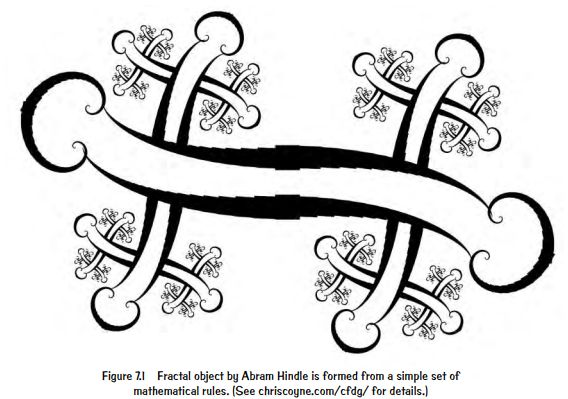## Universe is a swirl of equations

Our cosmos is not here by chance or it is not a random collection of matter and energy. Rather, Pickover says, it is formed through the application of mathematical principles or laws.

Although, initially, the universe could be a formless chaos. But eventually, it took a deliberate act of creation to bring it into being.

Hence, the universe is the result of a carefully crafted structure, which is governed by mathematical rules. Nothing is by-chance here.

## Probability is a subset of mathematics

Pickover then touches upon the topic of probability. With various examples, he tries to define what do we mean by the odds.

Probability, as per the author, is a tool for understanding uncertainty and predicting outcomes in situations where the outcome is also not certain.

He gives the reference of a spinning roulette wheel in a casino. And tries to evaluate the improbableness of our universe from a position where it’s already here.

To connect the two – randomness and mathematics – together, the author says that mathematics can contain elements of randomness and uncertainty. Or should I say, probability/ randomness/uncertainty is a subset of mathematics!

Although logics and well-defined paths of reasoning are the two strong foundations of mathematics, the reality is that, it can also involve unpredictable and probabilistic elements. Chaos theory, for instance, deal with complex systems that are inherently unpredictable and sensitive to small changes.

However, when it comes to conclusions, information coming from the funnel of probability will always lead to the likelihood of something happening, rather than its actual truth or validity.

Therefore, probability can never determine whether a particular conclusion or proposition is true or false. The truth of a proposition must be established via logical reasoning, empirical evidence, or direct observation, which only mathematics can provide.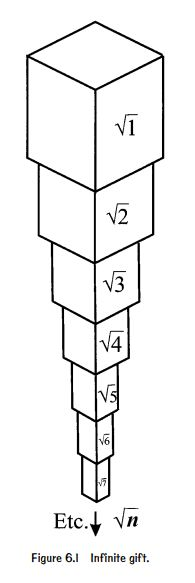## Infinity is a train on the landscape of reality

If the reality is a huge landscape, then infinity is like an endless train. And the train is trailing ceaselessly over it.

Tracks of the train denote the structure of the universe governed by the laws of physics. And the conductor of the train is none other than the intelligence that is operating behind the universe by creating and sustaining it.

The metaphor of “endless train” and “conductor” raises questions on deepest mysteries of existence, that is, what is the origin and nature of the universe?

Pickover suggests that since ages, mathematicians, philosophers, and mystics have been dealing with idea of the infinite. And he thinks the study of infinity can have a deeper meaning beyond its theoretical value.

## The mathematical theology

The quest to understand something that is beyond human comprehension is not only undertaken by mathematicians but similar feat is observed in mystical traditions as well. The latter call the quest – an attempt to understand God.

The difference lies in their approach. In mathematics, for instance, there are certain principles and methods, which are widely accepted, since every time, the steps are followed, they arrive at common solution.

Whereas in mystical traditions (or religions), beliefs are often based on faith and are not necessarily subject to verification or justification.

Religious beliefs are not put through empirical testing in the same way that mathematical theories are. And this highlights a key difference between the two fields.

## Science, mathematics, and arts are closely related

In the last section of the book, Pickover converges the three disciplines together. He suggests, science, mathematics, and arts have common ancestry. Both have their roots in ancient Greece.

All the three fields are based on a shared set of ideas and principles. Therefore, each cannot be studied in isolation but much can be gained from exploring the connections between them.

For instance, creative endeavors such as art when backed up by mathematical principles and algorithms can result in complex and intricate patterns. And the outcome is both beautiful and unpredictable. Thus, adding to the richness and diversity to the world of art.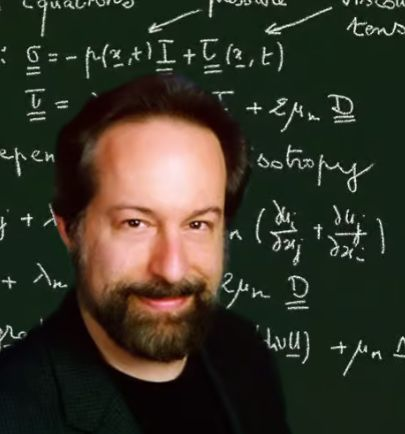## Takeaway

Passion for Mathematics by Clifford Pickover is one of the most profound works that I have read.

As a matter of fact, somewhere in between I almost forgot that I’m reading a book of mathematics. I thought that I’m ingesting some profound and deepest work in philosophy. So, if you want to understand or want to “see” the fabric of universe through the lens of numbers a.k.a. mathematics, this is the best book to start with. Highly recommended.